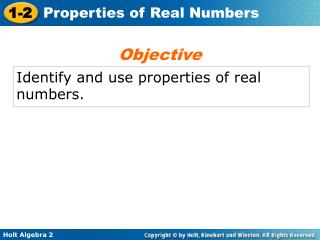# Identify and use properties of real numbers. - PowerPoint PPT PresentationDownload PresentationIdentify and use properties of real numbers.

Identify and use properties of real numbers.Download Presentation## Identify and use properties of real numbers.

- - - - - - - - - - - - - - - - - - - - - - - - - - - E N D - - - - - - - - - - - - - - - - - - - - - - - - - - -
##### Presentation Transcript

1. Objective Identify and use properties of real numbers.

2. The four basic math operations are addition, subtraction, multiplication, and division. Because subtraction is addition of the opposite and division is multiplication by the reciprocal, the properties of real numbers focus on addition and multiplication.

3. Properties Real Numbers Identities and Inverses

4. Properties Real Numbers Identities and Inverses

5. Properties Real Numbers Identities and Inverses

6. Properties Real Numbers Identities and Inverses

7. Recall from previous courses that the opposite of any number a is –a and the reciprocal of any nonzero number a is .

8. multiplicative inverse: The reciprocal of 12 is . Check Example 1A: Finding Inverses Find the additive and multiplicative inverse of each number. 12 additive inverse: –12 The opposite of 12 is –12. Check –12 + 12 = 0  The Additive Inverse Property holds. The Multiplicative Inverse Property holds.

9. The opposite of is . The reciprocal of is Example 1B: Finding Inverses Find the additive and multiplicative inverse of each number. additive inverse: multiplicative inverse:

10. The reciprocal of 500 is . multiplicative inverse: Check Check It Out! Example 1A Find the additive and multiplicative inverse of each number. 500 additive inverse: –500 The opposite of 500 is –500. Check 500 + (–500) = 0  The Additive Inverse Property holds. The Multiplicative Inverse Property holds.

11. Check It Out! Example 1B Find the additive and multiplicative inverse of each number. –0.01 additive inverse: 0.01 The opposite of –0.01 is 0.01. multiplicative inverse: –100 The reciprocal of –0.01 is –100.

12. Properties Real Numbers Addition and Multiplication

13. Properties Real Numbers Addition and Multiplication

14. Properties Real Numbers Addition and Multiplication

15. Properties Real Numbers Addition and Multiplication

16. Reading Math Based on the Closure Property, the real numbers are said to be closed under addition and closed under multiplication.

17. Example 2: Identifying Properties of Real Numbers Identify the property demonstrated by each question. Numbers are multiplied in any order without changing the results. A. 2  3.9 = 3.9  2 Commutative Property of Multiplication The numbers have been regrouped. B. Associative Property ofMultiplication

18. Check It Out! Example 2 Identify the property demonstrated by each equation. 2a. Numbers are multiplied in any order without changing the results. Commutative Property of Multiplication The numbers have been regrouped. 2b. 9(12) = (9  12) Associative Property of Multiplication

19. Think:5% = (10%) Example 3: Consumer Economics Application Use mental math to find a 5% tax on a \$42.40 purchase. Think:10% of \$42.40 Move the decimal point left 1 place. 10%(42.40) = 4.240 = 4.24 5% is half of 10%, so find half of 4.24. A 5% tax on a \$42.40 is \$2.12.

20. Check It Out! Example 3 Use mental math to find a 20% discount on a \$15.60 shirt. Think:20% = 10%  2 Move the decimal point left 1 place. 10%(15.60) = 1.560 = 1.56 1.56  2 = 3.12 20% is double 10%, so multiply 1.56 by 2. A 20% discount on a \$15.60 shirt is \$3.12.

21. Example 4A: Classifying Statements as Sometimes, Always, or Never True Classifying each statement as sometimes, always, or never true. Give examples or properties to support your answers. a b = a, where b = 3 True and false examples exist. The statement is true when a = 0 and false when a ≠ 0. sometimes true true example: 0 3 = 0 false example: 1 3 ≠ 1

22. Example 4B: Classifying Statements as Sometimes, Always, or Never True Classifying each statement as sometimes, always, or never true. Give examples or properties to support your answers. 3(a+ 1) = 3a + 3 Always true by the Distributive Property. always true

23. Check It Out! Example 4a Classify each statement as sometimes, always, or never true. Give examples or properties to support your answer. a + (–a) = b + (–b) Always true by the Additive Inverse Property.

24. Check It Out! Example 4b Classify each statement as sometimes, always, or never true. Give examples or properties to support your answer. a – (b + c) = (a – b) + (a – c) sometimes true True and false examples exist. The statement is true when a = 0, b = 1, and c = 2. False when a = 1, b = 2, and c = 3. true example: 0 – (1 + 2) = (0 – 1) + (0 – 2) –3 = –3 false example: 1 – (2 + 3) = (1 – 2) + (1 – 3) –4 ≠ –3